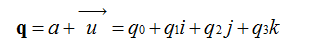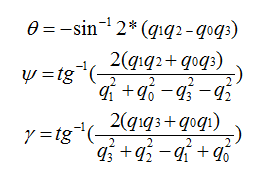# 硬件姿态解算

CrazePony使用了MPU6050自带的硬件四元数单元，可以通过IIC直接读取四元数，省却了软件解算繁琐的算法步骤，非常方便易用。norm = dmpinvSqrt(q*q + q*q + q*q + q*q);
q = q * norm;
q = q * norm;
q = q * norm;
q = q * norm;


DMP_DATA.dmp_roll = (atan2(2.0*(q*q + q*q), 1 - 2.0*(q*q + q*q)))* 180/M_PI;
// we let safe_asin() handle the singularities near 90/-90 in pitch
DMP_DATA.dmp_pitch = dmpsafe_asin(2.0*(q*q - q*q))* 180/M_PI;

DMP_DATA.dmp_yaw = -atan2(2.0*(q*q + q*q), 1 - 2.0*(q*q + q*q))* 180/M_PI;